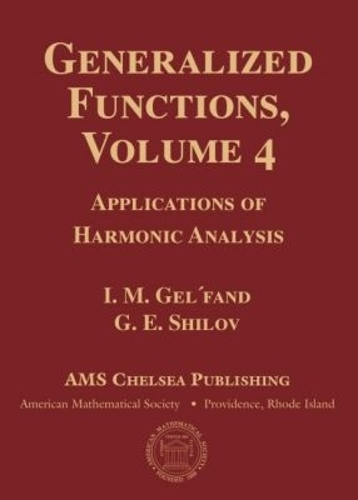•Generalized Functions, Volume 4: Applications of Harmonic Analysis - AMS Chelsea Publishing (Hardback)

(author), (author)
£49.50
Hardback 384 Pages / Published: 30/04/2016
• We can order this

Usually dispatched within 2 weeks

The first systematic theory of generalized functions (also known as distributions) was created in the early 1950s, although some aspects were developed much earlier, most notably in the definition of the Green's function in mathematics and in the work of Paul Dirac on quantum electrodynamics in physics. The six-volume collection, Generalized Functions, written by I. M. Gelfand and co-authors and published in Russian between 1958 and 1966, gives an introduction to generalized functions and presents various applications to analysis, PDE, stochastic processes, and representation theory.

The main goal of Volume 4 is to develop the functional analysis setup for the universe of generalized functions. The main notion introduced in this volume is the notion of rigged Hilbert space (also known as the equipped Hilbert space, or Gelfand triple). Such space is, in fact, a triple of topological vector spaces \$E \subset H \subset E'\$, where \$H\$ is a Hilbert space, \$E'\$ is dual to \$E\$, and inclusions \$E\subset H\$ and \$H\subset E'\$ are nuclear operators. The book is devoted to various applications of this notion, such as the theory of positive definite generalized functions, the theory of generalized stochastic processes, and the study of measures on linear topological spaces.

Publisher: American Mathematical Society
ISBN: 9781470426620
Number of pages: 384
Dimensions: 254 x 178 mm